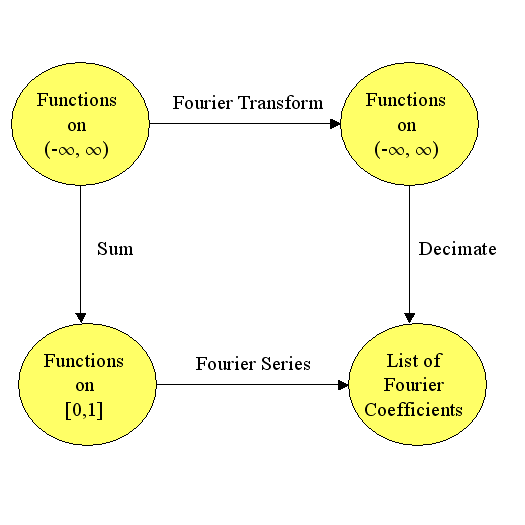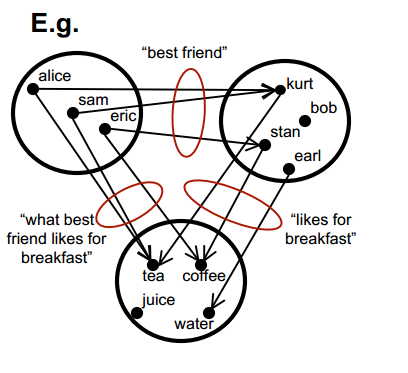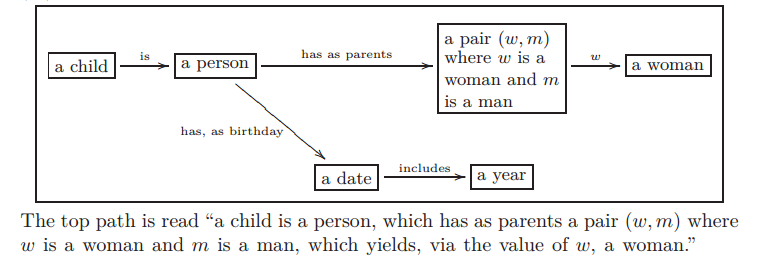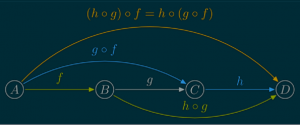# Category Theory

You are currently browsing the archive for the Category Theory category.

## Mathematical Concepts I use (almost) every week

So, I noticed Brian Rushton’s question on matheducators.stackexchange.com. He asked,

##### “What should be included in a freshman ‘Mathematics for computer programmers’ course?”

User dtldarek had a pretty cool reply broken up into three parts:

• Math that is actually useful.
• Math that you can run into, and is generally good to know.
• Math that lets you build more awesome math.

His answer got me to think about and revise my “100 Most useful Theorems and Ideas in Mathematics” post.  I wondered which of the 100 I used every week. Rather than trying to think about every week, I just thought it would be interesting to identify which ideas and theorems I used this week excluding the class I am teaching.

The first several ideas on the top 100 list, you can’t seem to avoid:  counting, zero, decimal notation, addition, subtraction, fractions, and negative numbers. Everyone uses these ideas when they buy anything or check their change.  (I wonder how many people rarely count the change from the cashier.)

The next group was mostly about algebra:  equality, substitution, variables, equations, distributive property, polynomials, and associativity.  Every week I attend two math seminars (Tensor Networks and Approximation Theory), so I use these ideas every week, just to add to the discussion during the seminar.

Strangely, I can’t remember using scientific notation this week except while teaching.  During class, I briefly mentioned the dinosaur killing asteroid (see the Chicxulub crater).  It’s hard to calculate any quantity about the asteroid (energy = 4 ×1023 Joules, mass = 2 ×1015 kg ?,  velocity $\approx$ 20000 meters/sec) without using scientific notation.  But, other than during class, I don’t remember using scientific notation.

During the Approximation Theory Seminar, we discussed polynomial approximations to the absolute value function so we used polynomials, infinity, limits, irrational numbers (square roots), the idea of a function, inequalities, basic set theory, vector spaces esp. Hilbert/Banach Spaces, continuity, Karush–Kuhn–Tucker conditions, linear regression, the triangle inequality, linearity, and symmetry during our discussion.  That seems like a lot, but when you have been using these ideas for decades, they are just part of your vocabulary.

So between the approximation theory seminar and daily restaurant mathematics, I used most of the first 40 items from the top 100 list.   Earlier in the week, I wrote up a simple category theory proof about isomorphisms and pull-backs.  Turns out, if you pullback an isomorphism and another arrow, then you get another isomorphism.  For example, in the commutative diagram below the top arrow and the bottom arrow are both isomorphisms (isometries even) and the entire diagram is a pullback.  If the bottom arrow is an isomorphism and the entire diagram is a pullback, then the top arrow is an isomorphism.  (The reverse is not true.)Working on the proof got me thinking about trig functions, modular arithmetic, injective/surjective functions, Fourier transforms, duality, eigenvalues, metric spaces, projections, the Reiz Representation Theorem, Plancherel’s theorem, and numerical integration.

Probability and information theory were largely missing from my week until I stopped by Carl’s house yesterday. He and I got into a discussion about category theory, information theory, and neural networks.  One thing we noticed was that 1-1 functions are the only functions that conserve the entropy of categorical variables. For example, if $X\in\{1,2,3,4,5,6\}$ is a die roll, then $Y=f(X)$ has the same entropy as $X$ only if $f$ is 1-1.  Turns out you can characterize many concepts from Category theory using entropy and information theory.  So we used random variables, probability distributions, histograms, the mean (entropy is the mean value of the log of the probability), standard deviations, the Pythagorean theorem, statistical independence, real numbers, correlation, the central limit theorem, Gaussian Distributions (the number e), integrals, chain rule, spheres, logarithms, matrices, conic sections (the log of the Gaussian is a paraboloid), Jacobians, Bayes Theorem, and compactness.

I almost avoided using the Pigeon Hole Principle, but then Carl argued that we could use the definition of average value to prove Pigeon Hole Principle.  I still don’t feel like I used it, but it did come up in a discussion.

Notably missing from my week were (surprisingly): derivatives, volume formulas (volume of a sphere or cube), Taylor’s theorem, the fundamental theorem of calculus, and about half of the ideas between #63 Boolean algebra and #99 uncountable infinity.

So, it looks like I typically use around 70 ideas from the list each week—more than I thought.

I will probably get back to 2048 stuff later this month and maybe a post on Fourier Transforms.

Have a great day!    – Hein

## Review: “Basic Category Theory for Computer Scientists”

So I have been wanting to understand Category Theory (see , , ) mainly because I thought it would help me understand advanced functional programming in Haskell and because of the book “Physics, topology, logic and computation: a Rosetta Stone” by Baez and Stay (2011).  A number of the constructs in Haskell were derived from Category Theory so that was enough motivation for me to learn it.  But also, monoidal categories are useful in several fields: physics, computer science, pure mathematics, and statistics. The biggest application for me is the idea of generalizing probabilistic graphical models through either Ologs or monoidal categories / tensor networks (see Brendan Fong’s thesis “Causal Theories: A Categorical Perspective on Bayesian Networks“).

To start my investigation of Category Theory, I began with the $20 thin book “Basic Category Theory for Computer Scientists” by Benjamin Pierce (see also the free online version “A taste of category theory for computer scientists” (1988)). I really enjoyed the book. Dr. Pierce’s style is a little informal compared to pure math books like Mac Lane’s “Categories for the Working Mathematician” (pdf / Amazon), but I enjoy that more relaxed style of writing when I am first learning a field. The biggest obstacle for learning category theory is the fact that category theory generalizes a lot of areas of pure mathematics like topology, abstract algebra, and geometry. It’s hard to generalize before you have examples to generalize, but the examples being generalized in category theory are mostly from higher level mathematics found in senior level undergraduate and graduate level courses. Pierce ameliorates this problem by introducing some of the most basic categories first: sets, ordered sets, partially ordered sets, groups, monoids, vector spaces, measure spaces, topological spaces, proofs, and a simple functional computer language. He takes the time to explicitly define most of these ideas, so, in theory, you could read this book without a background in theoretical mathematics, but it would be hard. After defining categories and introducing the most basic categories, Pierce describes and defines the most basic ideas in category theory: subcategories, commutative diagrams, monomorphisms, epimorphisms, isomorphisms, initial/terminal objects, products, coproducts, universal constructions, equalizers, pullbacks, pushouts, limits, cones, colimits, cocones, exponentiation, and closed Cartesian categories. These ideas are spelled out over the thirty pages of chapter one including illuminating homework exercises. The homework exercises varied significantly in difficulty. Many of the exercises were trivial and there are two or three that I am still working on despite investing several hours of thought. Generally, I found the exercises to be a bit harder than those in Mac Lane’s book, but Pierce’s book required less of a background in mathematics. A couple of the exercises were incorrectly stated or impossible. Chapter two introduced functors, natural transformations, adjoints, and F-algebras. After reading this chapter, I was finally able to understand the definition of monads which are an important part of the computer language Haskell! Pierce provides many examples of each of these ideas and enjoyable homework exercises to increase understanding. Pierce’s definition of adjoints is much easier to understand than the standard definitions using counit adjunction or Hom Sets. The last major chapter concerns applications of category theory to computer science–specifically lambda-calculus and programming language design. The first two chapters of the book give a reasonable condensed introduction to category theory for students that have taken a course in abstract algebra. A course in topology or linear algebra would be another useful prerequisite. I carried around the light 100 page book for a few months so that I could learn something whenever I had some extra time. I had hoped that when I had proven that several functors where monads, I would then really understand monads, but a full understanding still eludes me. Similarly, I have proven that several functor pairs are adjoint, but I still don’t feel as though I understand adjoint functors. I guess more work and learning are needed. Have a great year. – Cheers, Hein ## Six Notions of Abstraction So I have been learning a little category theory in the hopes that I could use it somehow to teach computers abstraction. What is abstraction? Carl and I have been talking about this for years. Here are some ideas on what abstraction could be: 1a) An average model of a large group of similar things. For example, the abstraction of a chair might be an idealized chair with a seat, a backrest, four legs, and possibly “strechers” between the legs to give it strength and stability. 1b) A function in a computer program that is used in several places in the code. In effect this function shortens the code. If the code has length L1 without the function, the code is length L2 with the function, the function is length LC, and the function is used N times in the code with the function, then typically L2$\approx\$ L1 – LC*N + LC + N.

The function is an abstraction of the pieces of code that it replaced similar to the way an ideal chair is an abstraction of many chairs.  The function has the common characteristics of the code it replaced.  For example, the following code consists of about 160 characters

// C Code without function

main()
{
printf("Hello John\n");
printf("Hello Harry\n");
printf("Hello Rose\n");
printf("Hello Carol\n");
printf("Hello Mr. Thomas\n");
printf("Hello Mrs. Jones\n");
}

and it could be shortened slightly to about 137 characters by

// C Code with function

void pr(char *s)
{
printf("Hello %s\n, s");
}

main() {
pr("John");
pr("Harry");
pr("Rose");
pr("Carol");
pr("Mr. Thomas");
pr("Mrs. Jones");
}

Though this example is not in any way practical, it does illustrate how a function can capture commonality.  All of the pieces of code replaced by the pr function had a call to printf, the substring “Hello “, and the carriage return “\n”.  The pr function was, in a sense, the idealized version of the six print statements in the original code.

In category theory, we could refer to the category of strings with arrows going from superstrings to substrings.  In this category, there would be an arrow from the line of code ”   printf(“Hello John\n”);” to the string ”   printf(“Hello \n”);”.  The categorical coproduct of several strings in this category would be the longest common substring.  So, category theory suggests the substring ”   printf(“Hello \n”);” should somehow be included in the abstraction of those six printf statements in the original code.

2)  A simplifying transformation that changes things into simpler things which are easier to work with.  For example, if you are counting sheep in a pen, you might convert groups of sheep into numbers and then add the numbers to get a total count for the entire pen.  We simplified the counting process by first converting groups of sheep into numbers.  Another example might be solving a corn maze by first flying over it, taking a picture, and then converting the “impassable” rows of corn into lines drawn on a piece of paper.  To solve the corn maze, you first convert it to a pen drawing on paper and then solve the pen drawn maze.

3)  Removing irrelevant information or detail.  If a piece in chess is attacking the opponent’s king, then the opponent must either a) move the king, b) put a piece between the attacker and the king, or c) capture the attacking piece.  If you wanted to list the possible moves for the opponent, you can often delete from the chess board most of the pieces because most of the pieces on the board do not affect the three options above.  In the diagram below, the white pawns, rook, and king and the black pawn on a7 do not affect the legal moves for black.Check

In a corn maze, the type of corn, the height of the corn stalks, the temperature, or the type of soil do not affect the solution of the corn maze, so that information could be ignored.  Ignoring irrelevant information allows the brain to form a more abstract, simple model of the corn maze.

4) Variables in first order predicate logic.  “If person A is taller than person B and person B is taller than person C, then person A is taller than person C.”  In that statement, person A could any one of a billion people on earth.

5) Establishing classes of objects.  “Fruit tends to spoil quickly.”  That statement applies to a huge variety of organic objects.  Abstraction classes can even be put in a hierarchy like   Animal ==>> Mammal ==> Cat ==> Siamese Cat.

6) Statistical Patterns and Compression.  If a piece of code like zip.exe compresses a corpus of English text files, we could say that the piece of code formed an abstract model of the corpus.  In the case of the zip function which uses the Lempel–Ziv–Markov chain algorithm, the zip program would notice that

• the letters ‘e’ and ‘t’ appear frequently,
• the letter ‘u’ usually follows ‘q’, and
• the capital letter I frequently appears with spaces on either side.

These patterns are all mini-abstractions or aspects of English.

A very general notion of abstraction could be any function which shortens the compression of data.  That function could be an abstraction of some part of the data.  If your data consisted of 3 dimensional models of chairs, then a compression program could use the fact that a chair usually has four legs, a seat, and a backrest.  A compression program might benefit from a function like the one shown below.

char *DescribeChair( double *featureVector)
{
char *description;

description = describeLegs(outputBuffer, featureVector);
description = describeSeatOnTopOfLegs(description,
featureVector);
description = describeBackRestOnTopOfSeat(description,
featureVector);

return description;
}

The abstract version of a chair would help compress the data describing many chairs.

A compression program for a corpus of chess games would probably include a board evaluation function.  The program could use the evaluation function to suggest the top five most likely moves.  This would allow the program to use less space to compress the moves by using a probability distribution (e.g. arithmetic encoding) over moves as a function of the “goodness” of each move.  Better moves would be more likely than worse moves.  Converting the positions to valuations helps the computer to compress by making it “understand” chess.  If the computer is compressing many chess games, it may even note that some human players were more aggressive players or stronger players.  By classifying the players by skill or behavior, the program achieves better compression.

Well that’s it for now.

Hopefully, sometime in the future, I will be describing how category theory can help us automate those six abstractions and then use the abstractions to generate features for learning algorithms.

(Code highlighting was done by http://hilite.me/.  Thanks!)

## “An introduction to Category Theory for Software Engineers”

Check out

An introduction to Category Theory for Software Engineers” by Steve Easterbrook.A category from “An introduction to Category Theory for Software Engineers” by Steve Easterbrook

## Knowledge Representation, Ologs, and Category theory

So I have been reading a number of texts in my quest to learn category theory and apply it to AI.  Two of the most interesting introductions to category theory are “Category Theory for Scientists” by Spivak (2013) and “Ologs: A Categorical Framework for Knowledge Representation” by Spivak and Kent (2012).

Ologs are a slightly more sophisticated, mathematical form of semantic networks.

Semantic networks are a graphical method for representing knowledge. Concepts are drawn as words (usually nouns) in boxes or ovals.  Relationships between concepts are depicted as lines or arrows between concepts.  (In graph theory, theses would be called edges.)  Usually, the lines connecting concepts are labeled with words to describe the relationship.  My favorite semantic network is the semantic network describing the bookGödel Escher Bach“, but here is a simpler example.

Mathematically, semantic networks are a graph where the nodes are concepts and the edges are relationships.  There are some cool algorithms for constructing semantic networks from text (see e.g. “Extracting Semantic Networks from Text via Relational Clustering” by Kok and Domingos (2008)).

Though semantic networks look like probabilistic graphical models (PGMs, a.k.a. Bayesian networks), they are different.  In PGMs, the nodes are random variables not general classes and if five edges point to a node, then there is an associated five parameter, real-valued function associated with those five edges indicating the likelihood that the target variable has a particular value.

As I said before, Ologs are a more sophisticated directed graph.  In Ologs, the nodes are typically nouns that can be assigned to one of many values.  In directed graphs the edge starts at one node called the tail and ends at another node called the head.  The edges in Ologs represent functions that given a value with the type of the tail node give you one value with the type of the head node. In the example shown below, the box “a date” could be assigned the values “Oct 12, 2010″ or “January 5, 2011″.  The corresponding values of the “a year” would be 2010 and 2011.Example Olog from “Ologs: A categorical framework for knowledge representation”

In category theory, categories are directed graphs where the edges are called arrows and they have some specific properties.  Ologs are part of the category Set.  One of the important properties of arrows is that if the head of one arrow is the tail of another arrow, then a third arrow is implied which is called the composition of the original two arrows.  In the diagram above the arrows “has, as birthday” and “includes” can be combined to create a new arrow from “a person” to “a year” which assigns to each person his or her birth year.

I just wanted to introduce categories and Ologs for later articles.

Cheers – Hein

## Yann Esposito’s Category theory Slides with Haskell

I really enjoyed Yann Esposito’s slides “Category Theory Presentation” which give a relatively simple and artistic introduction to category theory for Haskell programmers.  (I like Haskell too.)  His slides present the basic definitions of categories, functors, natural transformations, and monads along with working Haskell code.

Here is a fragment of a typical slide.## Category Theory ?

Artificial intelligence and machine learning are linked with a huge list of other fields of study: statistics, probability, information theory, computational complexity theory, algorithmic complexity theory, linear regression, linear programming, approximation theory, neuroscience, automata theory, geometry of high dimensional spaces, reproducing kernal Hilbert spaces, optimization, formal languages, and many other areas (see e.g. the graphic below by Swami Chandrasekaran).

My focus has always been on applied mathematics rather than “pure” mathematics. But, by studying AI I was almost forced into learning about areas that I had avoided in graduate school like decidability (logic) and proof theory which did not seem practical at the time.  I felt that most abstract mathematics and some abstract computer science, though beautiful, were not very useful. So, I am surprised that I am now studying the most abstract and possibly most useless area of mathematics, Category Theory.

Why category theory (aka “Abstract Nonsense“)?  Mathematicians often wonder “What is the simplest example of this idea?”, “Where do idea A and idea B intersect?”, and “How does this idea generalize?”.  Category theory seems to be about theses questions which I hope will be useful for AI research.  I was also inspired to read about category theory by Ireson-Paine‘s article “What Might Category Theory do for Artificial Intelligence and Cognitive Science?” and “Ologs: A Categorical Framework for Knowledge Representation” by Spivak and Kent (2012). Paine describes relationships between category theory, logical unification, holographic reduced representation, neural nets, the Physical Symbol System Hypothesis, analogical reasoning, and logic. The article includes many authoritative interesting references and links.  Ologs appear to be a category theoretic version of semantic networks.

Since I am studying category theory in my off time, I will probably blog about it. I am an enthusiastic beginner when it comes to category theory, so hopefully I can communicate that enthusiasm and avoid posting the common conceptual blunders that are part of learning a new field.

Cheers, Hein

PS:  I loved this graphic by Swami Chandrasekaran from the article “Becoming a Data Scientist“.